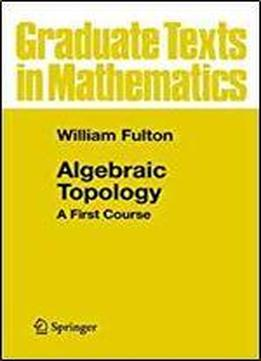Algebraic Topology: A First Course (graduate Texts In Mathematics) by William Fulton / 1997 / English / DjVu

To the Teacher. This book is designed to introduce a student to some of the important ideas of algebraic topology by emphasizing the re­ lations of these ideas with other areas of mathematics. Rather than choosing one point of view of modem topology (homotopy theory, simplicial complexes, singular theory, axiomatic homology, differ­ ential topology, etc.), we concentrate our attention on concrete prob­ lems in low dimensions, introducing only as much algebraic machin­ ery as necessary for the problems we meet. This makes it possible to see a wider variety of important features of the subject than is usual in a beginning text. The book is designed for students of mathematics or science who are not aiming to become practicing algebraic topol­ ogists-without, we hope, discouraging budding topologists. We also feel that this approach is in better harmony with the historical devel­ opment of the subject. What would we like a student to know after a first course in to­ pology (assuming we reject the answer: half of what one would like the student to know after a second course in topology)? Our answers to this have guided the choice of material, which includes: under­ standing the relation between homology and integration, first on plane domains, later on Riemann surfaces and in higher dimensions wind­ ing numbers and degrees of mappings, fixed-point theorems appli­ cations such as the Jordan curve theorem, invariance of domain in­ dices of vector fields and Euler characteristics fundamental groups

views: 435